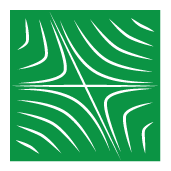# Dynamical Systems at the University of Utah

In mathematics, a dynamical system is a system in which a function describes the time dependence of a point in a geometrical space. Examples include the mathematical models that describe the swinging of a clock pendulum, the flow of water in a pipe, and the number of fish each springtime in a lake.### Vector Fields Plot

The vector field v(x) is a smooth function that at every point of the phase space M provides the velocity vector of the dynamical system at that point.### Systems 'n Coffee

Systems 'n Coffee is a bimonthly reading group committed to interdisciplinary research using Dynamical Systems Theory.### Quantitative Reasoning Under a Dynamical Social Science

by Dr. Jonathan Butner